# Frames, ticks, titles, and labels

Setting the style of the map frames, ticks, etc, is handled by the `frame` parameter that all plotting methods of `pygmt.Figure`.

```import pygmt
```

## Plot frame

By default, PyGMT does not add a frame to your plot. For example, we can plot the coastlines of the world with a Mercator projection:

```fig = pygmt.Figure()
fig.coast(shorelines="1/0.5p", region=[-180, 180, -60, 60], projection="M25c")
fig.show()
```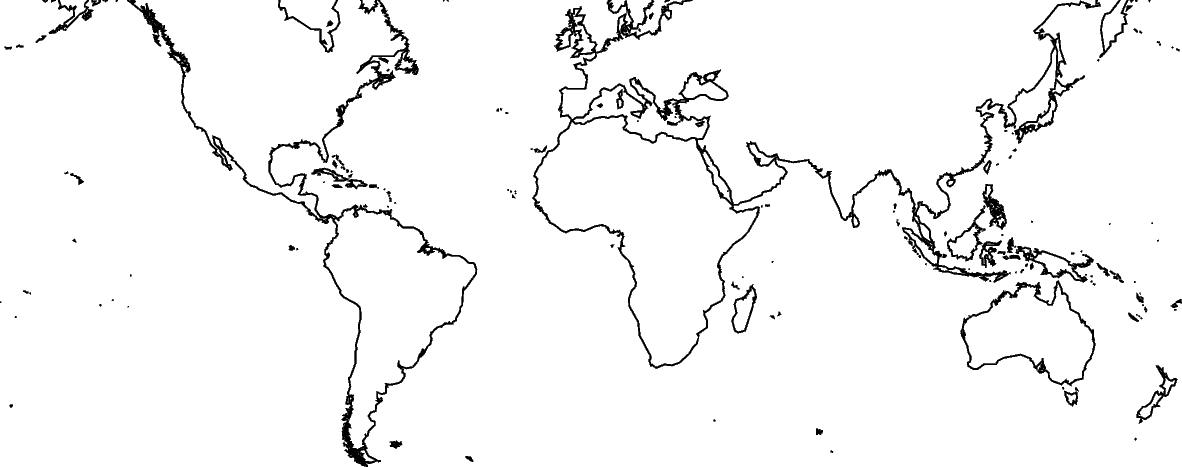Out:

```<IPython.core.display.Image object>
```

To add the default GMT frame to the plot, use `frame="f"` in `pygmt.Figure.basemap` or any other plotting method:

```fig = pygmt.Figure()
fig.coast(shorelines="1/0.5p", region=[-180, 180, -60, 60], projection="M25c")
fig.basemap(frame="f")
fig.show()
```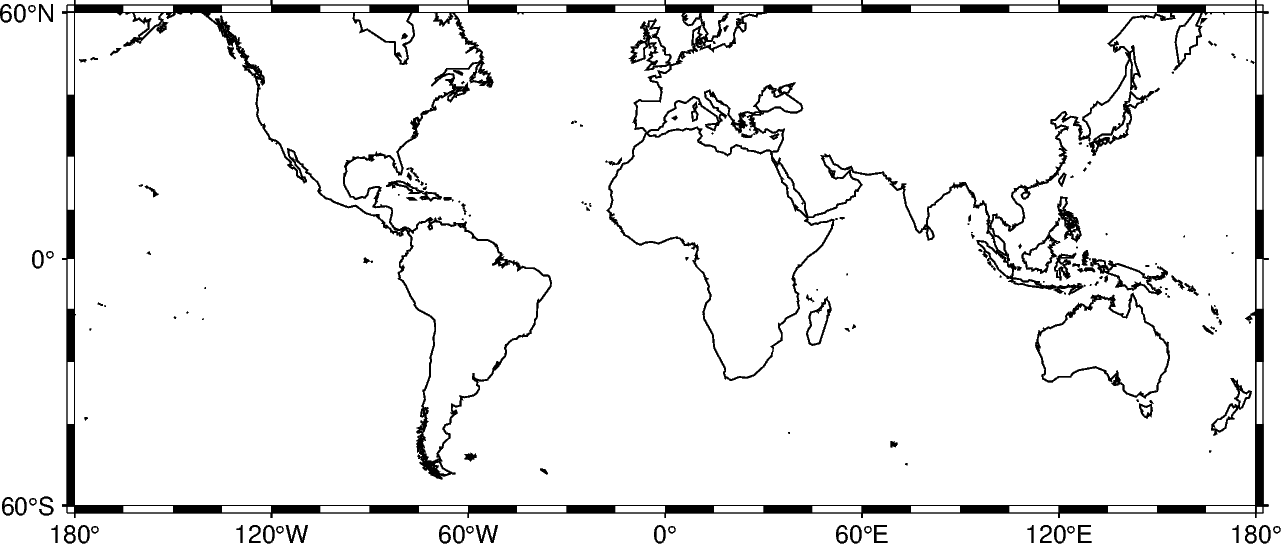Out:

```<IPython.core.display.Image object>
```

## Ticks and grid lines

The automatic frame (`frame=True` or `frame="a"`) sets the default GMT style frame and automatically determines tick labels from the plot region.

```fig = pygmt.Figure()
fig.coast(shorelines="1/0.5p", region=[-180, 180, -60, 60], projection="M25c")
fig.basemap(frame="a")
fig.show()
```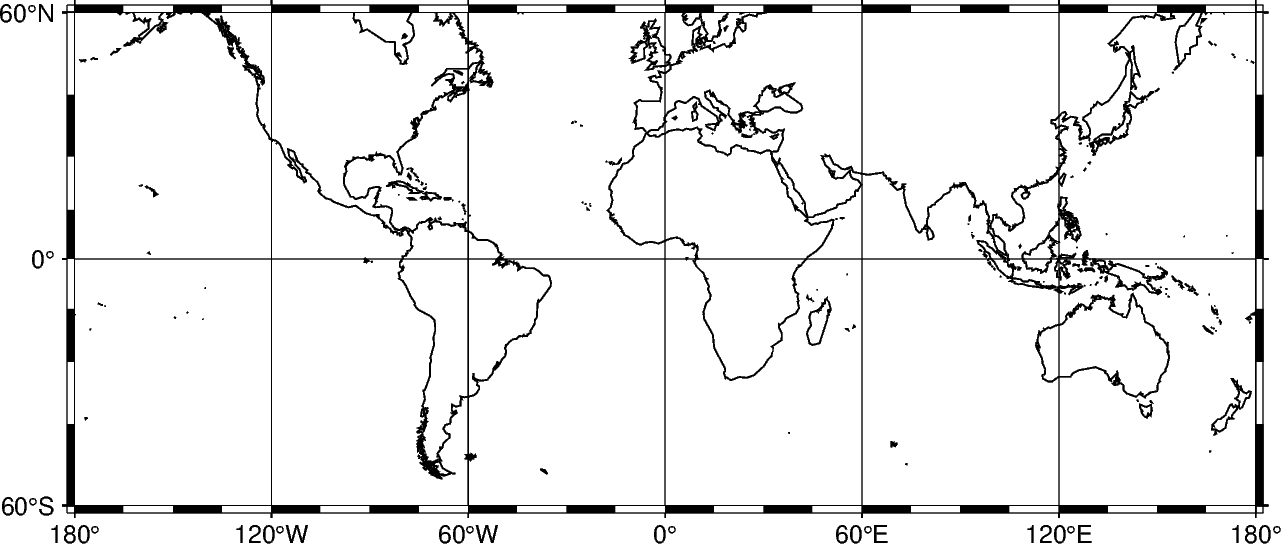Out:

```<IPython.core.display.Image object>
```

Add automatic grid lines to the plot by adding a `g` to `frame`:

```fig = pygmt.Figure()
fig.coast(shorelines="1/0.5p", region=[-180, 180, -60, 60], projection="M25c")
fig.basemap(frame="ag")
fig.show()
```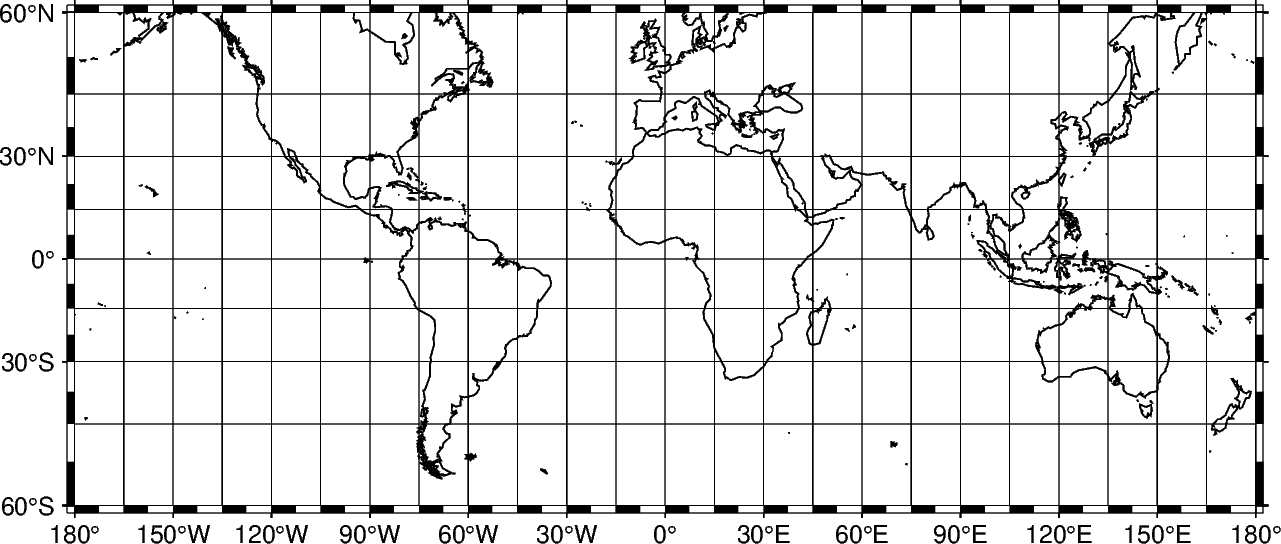Out:

```<IPython.core.display.Image object>
```

## Title

The figure title can be set by passing +ttitle to the `frame` parameter of `pygmt.Figure.basemap`. Passing multiple arguments to `frame` can be done by using a list, as show in the example below.

```fig = pygmt.Figure()
# region="IS" specifies Iceland using the ISO country code
fig.coast(shorelines="1/0.5p", region="IS", projection="M25c")
fig.basemap(frame=["a", "+tIceland"])
fig.show()
```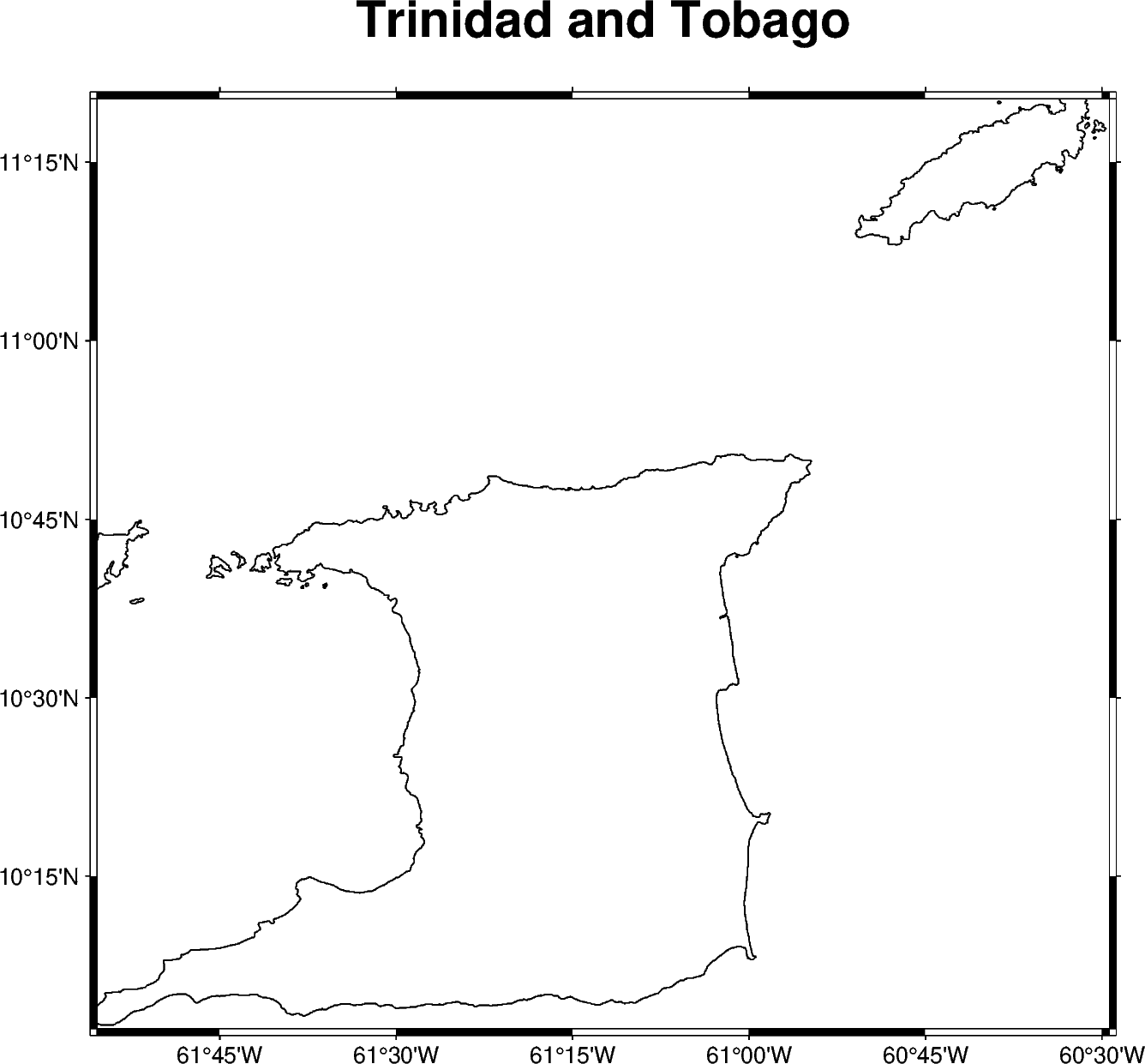Out:

```<IPython.core.display.Image object>
```

To use a title with multiple words, the title must be placed inside another set of quotation marks. To prevent the quotation marks from appearing in the figure title, the `frame` parameter can be passed in single quotation marks and the title can be passed in double quotation marks.

```fig = pygmt.Figure()
# region="TT" specifies Trinidad and Tobago
fig.coast(shorelines="1/0.5p", region="TT", projection="M25c")
fig.show()
```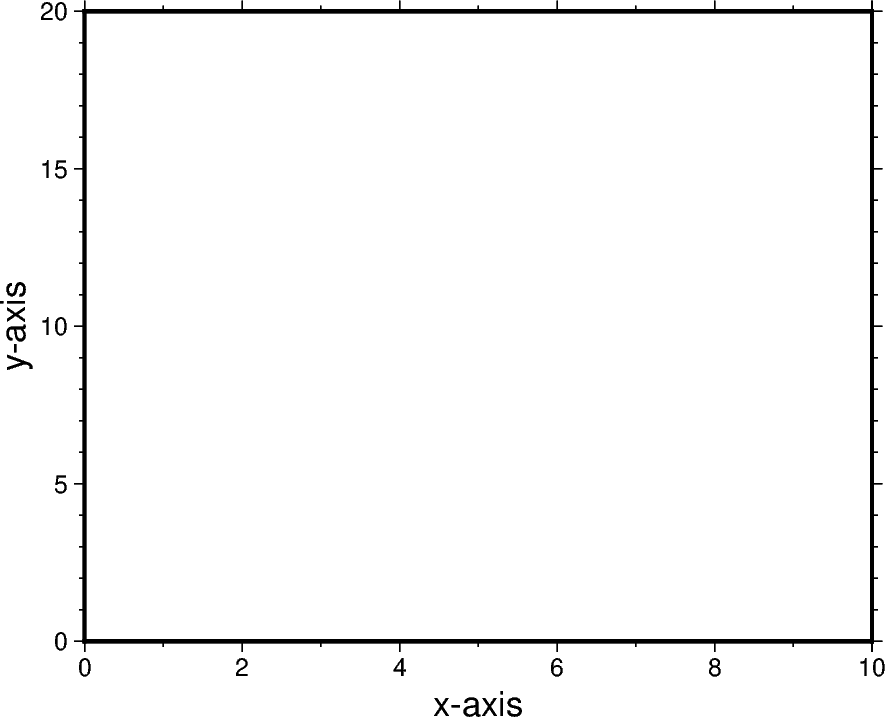Out:

```<IPython.core.display.Image object>
```

## Axis labels

Axis labels can be set by passing x+llabel (or starting with y if labeling the y-axis) to the `frame` parameter of `pygmt.Figure.basemap`. By default, all 4 map boundaries (or plot axes) are plotted with both tick marks and axis labels. The axes are named as W (west/left), S (south/bottom), N (north/top), and E (east/right) sides of a figure. If an upper-case axis name is passed, the axis is plotted with tick marks and axis labels. A lower case axis name plots only the axis and tick marks.

The example below uses a Cartesian projection, as GMT does not allow axis labels to be set for geographic maps.

```fig = pygmt.Figure()
fig.basemap(
region=[0, 10, 0, 20],
projection="X10c/8c",
# Plot axis, tick marks, and axis labels on the west/left and south/bottom
# axes
# Plot axis and tick marks on the north/top and east/right axes
frame=["WSne", "xaf+lx-axis", "yaf+ly-axis"],
)
fig.show()
```Out:

```<IPython.core.display.Image object>
```

Total running time of the script: ( 0 minutes 8.016 seconds)

Gallery generated by Sphinx-Gallery